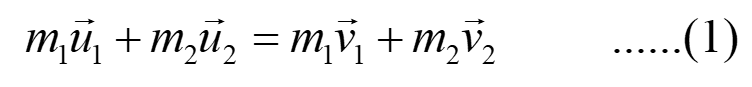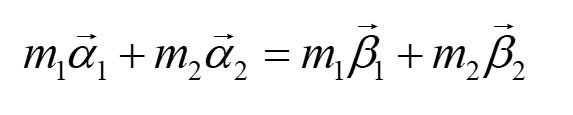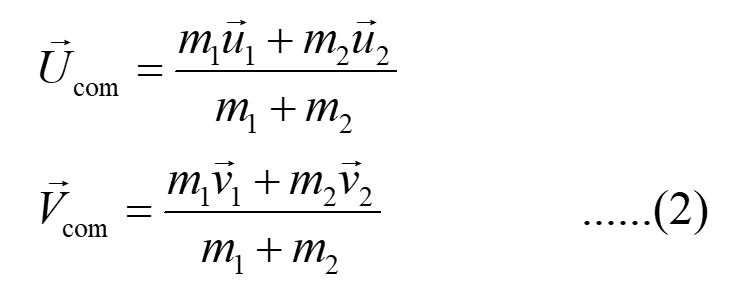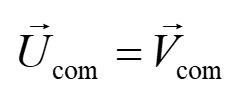# How are various quantities related in laboratory and Center of mass frames?

Question
1 views

How are various quantities related in laboratory and Center of mass frames?

check_circle

Step 1

The frame in which the quantities like position and velocities are measured wrt the laboratory is known as the laboratory frame.

In this frame, the laboratory is at rest and all the experiment is performed within this frame.

Consider an example of conservation of momentum in the laboratory frame.Here, u1 and u2  are the initial velocity of the first and the second particle respectively.

v1 and v2  are the final velocity of the first and the second particle respectively.

All the velocities are measured wrt the lab.

Step 2

The frame in which the quantities like position and velocities are measured wrt the COM (center of mass) of the system is known as COM frame.

Consider an example of conservation of momentum in the COM frame.Here, α1 and α2 are the initial velocities in the COM frame of the first and the second particle respectively.

β1 and β2 are the final velocities in the COM frame of the first and the second particle respectively.

The initial velocity (Ucom) and the final velocity (Vcom) of the COM can be determined as,From equation (1) and (2), we get,This implies the velocity of the COM is unchanged/unaltered.

...

### Want to see the full answer?

See Solution

#### Want to see this answer and more?

Solutions are written by subject experts who are available 24/7. Questions are typically answered within 1 hour.*

See Solution
*Response times may vary by subject and question.
Tagged in